# Average velocity (constant acceleration) Formula

Average velocity (constant acceleration) Formula

Velocity is the rate at which an object moves. It has both a magnitude (a value) and a direction. When a velocity is changing as a result of a constant acceleration, the average velocity can be found by adding the initial and final velocities, and dividing by 2. The unit for velocity is meters per second (m/s). Note that this formula applies for constant acceleration only.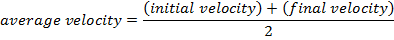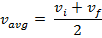vavg = average velocity (m/s)

vi = the initial velocity (m/s)

vf = the final velocity (m/s)

Average velocity (constant acceleration) Formula Questions:

1) A truck is travelling forward at a constant velocity of 10.00 m/s, and then begins accelerating at a constant rate. A short time later, the velocity of the truck is 30.00 m/s, forward. What was the average velocity of the truck during its acceleration?

Answer: The initial velocity is vi = 10.00 m/s, in the forward direction. The final velocity is vf = 30.00 m/s in the forward direction. The average velocity during the acceleration can be found using the formula: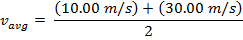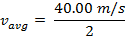vavg = 20.0 m/s

The average velocity during the truck's acceleration was 20.0 m/s, forward.

2) A child throws a ball in the air. The ball leaves her hand with a velocity of 2.00 m/s upward. The ball experiences acceleration due to gravity, and after a short time, the velocity of the ball is 2.00 m/s downward. What is the average velocity of the ball?

Answer: If the positive direction is defined to be "up", then the initial velocity vi = +2.00 m/s, and the final velocity vf = -2.00 m/s. The average velocity of the ball can be found using these values and the formula: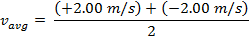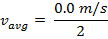vavg = 0.0 m/s

The average velocity of the ball within the time interval was 0.0 m/s.